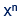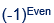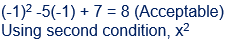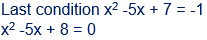# The number of integers that satisfy the equality - Quantitative Aptitude - CAT Quadratic Equation

## CAT 2020 - Slot 2 - Quantitative Aptitude - Quadratic Equation - Question 17 - The number of integers that satisfy the equality

Q. 17: The number of integers that satisfy the equality (x2 – 5x + 7)x + 1 = 1 is

##### 1. 52. 43. 34. 2

We can have three possibilities for the equality of form= 1

First n = 0, then x = 1 and then x = -1 and n = evenUsing first x = -1, and substituting in given equationSolving x = 2 or x = 3 (Both are acceptable)No integer values,

So 3 values satisfy the equality

### Past Year Question Paper & SolutionsCounselling Session
By IIM Mentor

#### Free Material Area